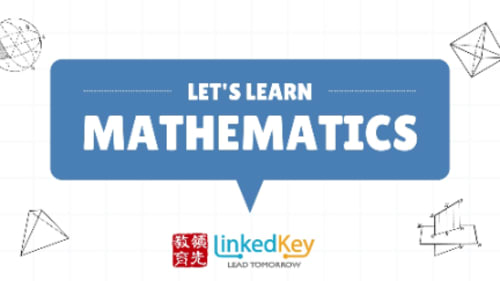### Interested in: Math Curriculum Course For G5-12

Fields marked with an asterisk (*) are required

# Math Curriculum Course For G5-12• Program
• Age 10 - 18 (Coed)
• Specialized in STEM and Math—offers 3 activities
• 1 session date to choose from
• Cost starting from \$240
• Busing not available
• Special needs support not available

Course Intro

This course will support students’ success at their day school. It is also for students who want to pre-learn math curriculum to stay ahead of time. We have content covering all G5-12 curriculum and practicing problem-solving skills. It’s a way not only to improve students’ scores but also to lay the foundation for future math learning. Classes are 2 hours long and will have homework after each class.*We Provide both In-Person & Online Classes. When students completed the curriculum classes, we'll recommend them to participate different math contests according to their level.

Math G3-10 Curriculum, AP & IB Math

• G7 Math Curriculum
• Number Part 1: Whole numbers
Number Part 2: Fractions
Number Part 3: Decimals
Number Part 4: Orders of Operations-key concepts and examples
Number Part 5: Relating Fractions, Decimals, and Percents
Number Part 6: Exploring rates and ratios
Number Part 7: Exploring Integers
Algebra: Solving equations and coding
Financial Literacy: Money Concepts
Financial Literacy: Financial Management, Consumer and Civic Awareness
Spatial Sense: Measurement
Spatial Sense: Surface Area and Volume of Polyhedron
Spatial Sense: Location and Movement
Data: Data Literacy
Data: Probability
• G8 Math Curriculum
• 1. Number Sense Properties and Relationships
2. Algebra Patterns and Relationships
3. Equations and Inequalities
4. Coding Coding Skills
5. Data Literacy, Collection, Organization and Visualization
6. Probability, theoretical and experimental probabilities
7. Spatial Sense, Geometric and Spatial Reasoning, Measurement
8. Financial Literacy , Money and Finances, Financial Management
9. Miscellaneous questions
• G9 Math Curriculum
• 1. Mathematical Processes
2. Relations
3. Polynomials
4. Equations
5. Modelling With Graphs
6. Analyse Linear Relations
7. Geometric Relationships
8. Measurement Relationships
9. Optimizing Measurements
• G10 Math Curriculum
• 1. Linear systems
2. Analytic geometry
3. Geometric properties
7. Trigonometry of right triangles
8. Trigonometry of acute triangles
• Functions G11
• 1. Introduction to Functions
2. Equlvalent Algebraic Expressions
4. Exponential Functions
5. Trigonometric Ratios
6. Sinusoidal Functions
7. Discrete Functions: Sequences and Series
8. Discrete Functions: Financial Applications
• Data Management (for G11 students who will take AP Statistics Exam)
• 1.Probability Theorem, Venn Diagram (1)
2.DeMorgan’s Law, Conditional Probability (1)
3.Independent and Dependent Events (1)
4.Fundamental Counting Principle, Permutations (1)
5.Combinations, Problem Solving (2)
6.One-Variable Statistics (2)
7.Binomial Theorem, Intro to Probability Distributions (2)
8.Binomial Distribution, Geometric Distribution, Hypergeometric Distribution (1-2)
9.Normal Distributions (2)
10.Two-Variable Stats, Sampling and Bias
• 1. Polynomial Functions
2. Polynomial Equations and Inequalities
3. Rational Functions
4. Trigonometry
5. Trigonometric Functions
6. Exponential and Logarithmic Functions
7. Tools and Strategies for Solving Exponential and Logarithmic Equations
8. Combing Functions
• Calculus & Vectors G12
• 1. Limits and continuity of function (4h)
2. Derivative and corresponding rules (4h)
3. Derivative of exponential and trigonometric functions (3h)
4. Application of derivatives (3h)
5. Sketch the curve of functions (6h)
6. Definition of vector and operation between vctors(3h)
7. Dot product and cross product of vectors(3h)
8. Equation of lines and planes(6h)
• University Math (Calculus, Vectors in Year 1)

LinkedKey Mississauga Campus: 3300 Ridgeway Dr #11, Mississauga, ON

### Session Dates and Rates

 Sep 05. '23 - Jan 14. '24 Mississauga, ON 3300 Ridgeway Dr. Unit 11 09:00 - 21:15 S M T W T F S \$240 - \$960 2023-09-05 240

### Activities

Recreational         Instructional
Intense or Competitive

Math
STEM

### Contact

Math Curriculum Course For G5-12 is run by LinkedKey.

### Interested in:

Fields marked with an asterisk (*) are required
Date:
Location:Our Kids: The Trusted Source for Families since 1998.

Thank you for using OurKids.net.